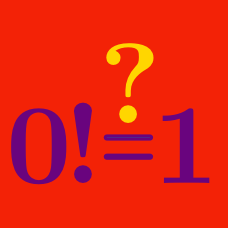Number Theory

# Number Theory Common Misconceptions: Level 2 Challenges

What is the smallest prime number?

What is the value of $\Large 0!$

True or false:

The sum of two irrational numbers is always irrational.

$\Large \color{#D61F06}{A} - \color{#3D99F6}{B} = \color{#D61F06}{A} \div \color{#3D99F6}{B}$

If whole numbers $\color{#D61F06}{A}$ and $\color{#3D99F6}{B}$ satisfy this equation, then what is $\color{#D61F06}{A} + \color{#3D99F6}{B}?$

Bonus: Can you explain why there is only one solution?

Which of these statements are true?

(A). "$1$ is a prime number"

(B). "$0$ is neither even nor odd"

(C). "$\sqrt{x^2}$ is always equal to $x$"

(D). "$\dfrac{1}{4}$ is neither even nor odd"

×